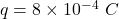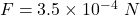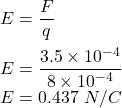## A positive charge of 8.0 × 10-4 C is in an electric field that exerts a force of 3.5 × 10-4 N on it. What is the strength of the electric fi

Question

A positive charge of 8.0 × 10-4 C is in an electric field that exerts a force of 3.5 × 10-4 N on it. What is the strength of the electric field?

in progress 0
2 months 2021-07-29T21:03:45+00:00 1 Answers 4 views 0

E = 0.437 N/C

Explanation:

Given that,

Charge,Electric force,Let the strength of the electric field is E. We know that, the electric force is given by :

F = qE

Where

E is the electric field strengthSo, the strength of the electric field is equal to 0.437 N/C.